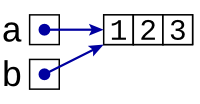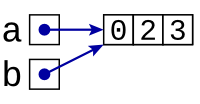# References & parameters

What do you suppose will the following program display?

```a = 1 b = a b = 0 print(a) ```

The first line sets `a` to 1, the second copies that 1 into `b`, the third sets `b` to 0, and the fourth displays `a`. At this point, `a` is still 1: When we executed the statement “`b = a`”, the value of `a` is copied into `b`, but Python forgets any link to `a` beyond that, so the later change to `b` doesn't alter `a`. So this short program displays the value 1.

The following program ends up being essentially equivalent:

```def set_to_zero(b):  # (Function's name is misleading!)     b = 0 a = 1 set_to_zero(a) print(a) ```

When we call “`set_to_zero(a)`”, again, the value of `a` — that is, 1 — is copied into the parameter variable `b`. Just as before, no long-term link between `b` and `a` exists, so the assignment to `b` within `set_to_zero` only changes `b`, not `a`. Once we return from `set_to_zero`, then, we end up displaying the current value of `a`, which is still 1.

(Despite its name, the `set_to_zero` function is completely pointless: All it does is set the value of its parameter variable, a variable that is promptly lost as soon as we return from the function.)

Now let's look at another little program.

```a = [1, 2, 3] b = a b = 0 print(a) ```

This program behaves very differently: Variables don't hold lists in the same sense that they hold numbers. After all, the “box” corresponding to a variable has a fixed size, whereas a list often takes up a large amount of memory. Instead, a variable actually holds a reference to a list. We can diagram this with a picture, using an arrow in `a`'s box pointing to the list.In the assignment statement “`b = a`”, we copy the contents of `a`'s “box” into `b`'s “box”. In this case, `a`'s box just contains an arrow, so an arrow to the same location is placed in `b`'s box.Once you see this picture of `a` and `b` both referencing the same list, you naturally expect that the line &lqduo;`b = 0`” to change that shared list.Consequently, in displaying `a`, we will end up displaying the value 0.

Looking at the following program, then, you will naturally expect the behavior to be the same as before.

```def set_to_zero(b):     b = 0 a = [1, 2, 3] set_to_zero(a) print(a) ```

In calling “`set_to_zero(a)`”, Python will make `b` reference the same list as `a` does. Then `set_to_zero` alters the contents of that list before returning, so that `a` will end up being 0.

Suppose, however, we had written the following.

```def set_to_zero(b):     b = [0, 2, 3] a = [1, 2, 3] set_to_zero(a) print(a) ```

This is entirely different. Rather than change the list that `b` is referencing, the line “`b = [0, 2, 3]`” leads `b` to reference another list altogether.(after `a = [1, 2, 3]`)(after entering `set_to_zero(a)`)(after `b = [0, 2, 3])`

Consequently, in displaying `a`, this program ends up displaying 1.